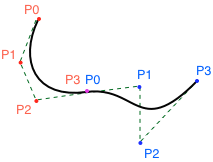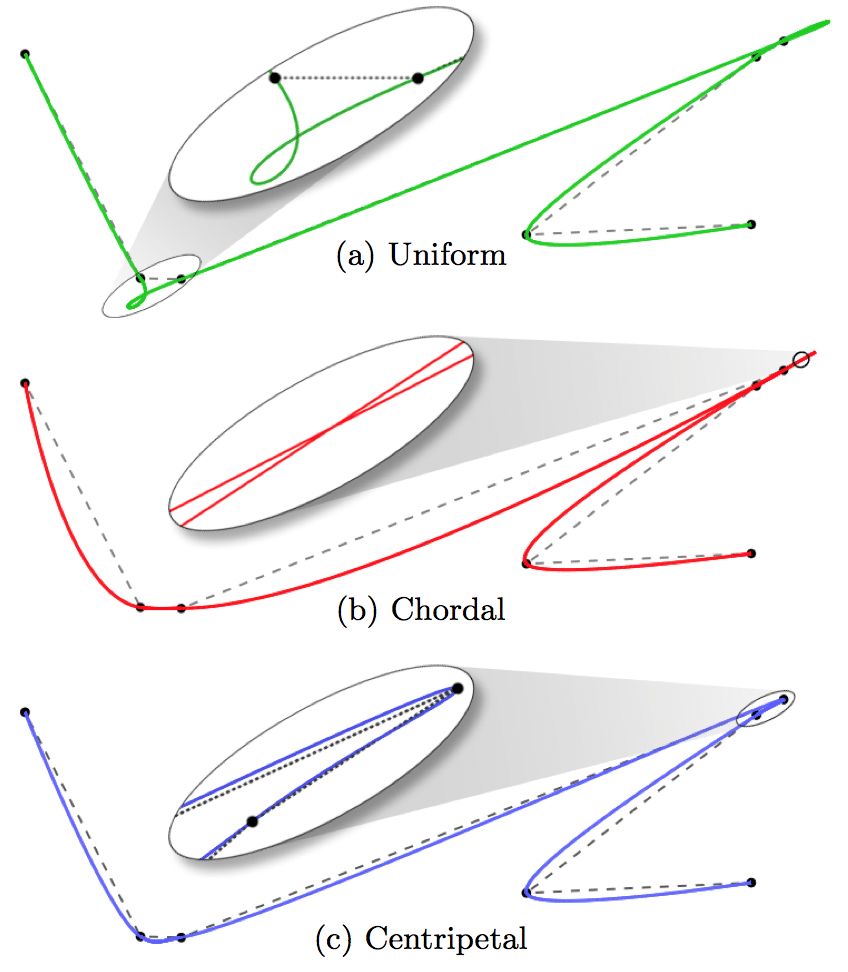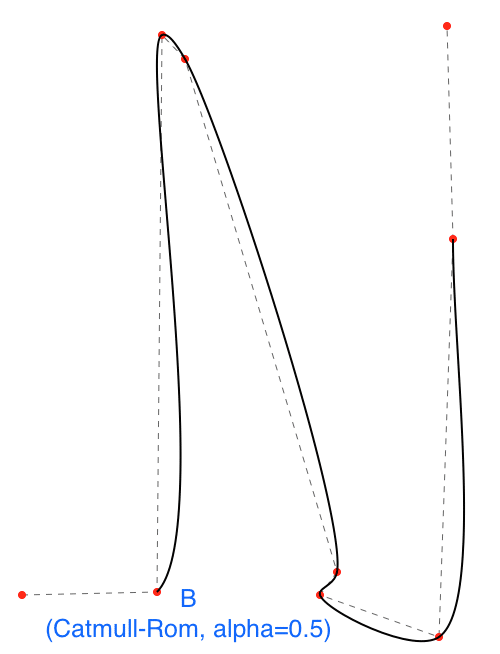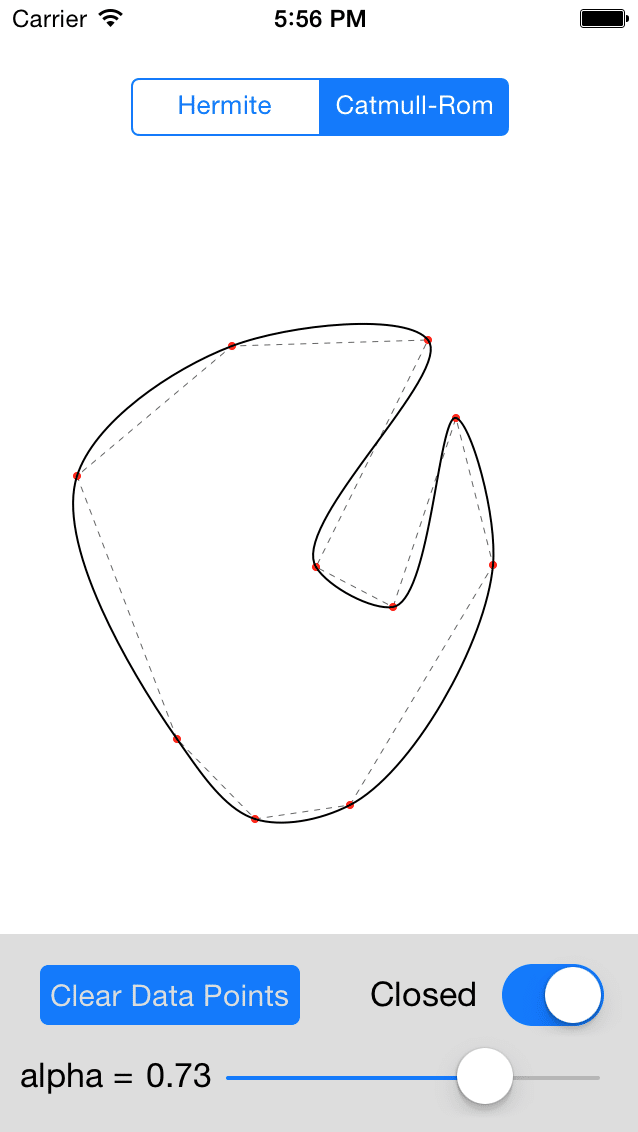# Interpolating Points in iOS with UIBezierPath

### Article summaryRecently, I have been working on a mobile app that includes a visualization consisting of smooth curves passing through arbitrary, changing sets of 2D points. The most straightforward way to accomplish this in iOS is using a string of smooth curves defined in a UIBezierPath, however it is up to the developer to construct the cubic bezier curves in the path in such a way that they are smooth and pass exactly through the data.

In this post I’ll describe two simple and commonly-used methods for interpolating points with cubic Bezier curves (without diving into the math behind them), and also link to a git repo I wrote that includesan implementation of the two interpolation methods.

## UIBezierPath and Bezier Curves

In iOS, we draw segments of straight and curved line segments using UIBezierPath. The linear segments are very easy to add via `addLineToPoint:`, but what about a curved shape?

Curved segments can drawn by adding cubic Bezier curves in the path. Cubic Bezier curves are defined by four control points- the placement of these four points define the curve’s shape. In the picture below, each point is a 2D (x,y) point in a Euclidean space.Adding Bezier curves to a UIBezierPath is straightforward:

```UIBezierPath* bezierPath = [UIBezierPath bezierPath];
[bezierPath moveToPoint: CGPointMake(77.5, 36.5)];
[bezierPath addCurveToPoint: CGPointMake(101.5, 72.5) controlPoint1: CGPointMake(67.78, 56.83) controlPoint2: CGPointMake(75.76, 76.01)];
[bezierPath addCurveToPoint: CGPointMake(157.5, 66.5) controlPoint1: CGPointMake(127.24, 68.99) controlPoint2: CGPointMake(127.69, 97.13)];
```

In the above code, the UIBezierPath starts at (77.5, 36.5), and two cubic Bezier curves are added using `addCurveToPoint:controlPoint1:controlPoint2`. Here’s what the two curves will look like:I have marked the four control points of the first curve (C1) in red and the four control points of the second curve (C2) in blue. Both curves share a control point (C1 P3 and C2 P0). C1’s first control point corresponds to the start of the UIBezierPath at P0=(77.5, 36.5), its first control point at (67.78, 56.83) corresponds to P1, and so on.

Clearly, the trivial choice of the positions of both curves endpoints (P0 and P3) will force the curves to pass exactly through two interpolation points. It follows that for a set of N points, we can create N-1 curves that pass through every point. For the transition between two adjacent curves to be smooth, the adjacent control points (P2 in the first curve, and P1 in the second) must be at least co-linear, and ideally we would also like them to have the same length. If they are not co-linear, the UIBezierPath will have cusps. The problem is, how do we position these internal control points?

## Smooth Interpolation Using Hermite and Catmull-Rom Splines

Two of the most commonly used interpolating curves are Hermite and Catmull-Rom splines. These curves are defined by the set of interpolating points and both are readily converted to a set of piecewise cubic Bezier curves — meaning that given N fitting points, we can create the control points for N-1 cubic Bezier curves that match the Hermite or Catmull-Rom splines. The cubic Beziers are then added to a UIBezierPath.

The simplest approach to interpolating is probably using cubic Hermite splines. Computing the corresponding cubic Bezier control points for a Hermite curve is very simple (see code linked below in the example project), but they exhibit problems such as extremely high curvature “kinks” and loops when points are irregularly spaced:Both curves A and B are created by Hermite interpolation. Curve A looks good — the points are roughly uniformly spaced. Curve B, however, has kinks and self-intersections due to the irregularly-spaced points.

Another option for fitting points with curves is using Catmull-Rom spline curves. Like Hermite curves, Catmull-Rom curves will pass through the interpolation points and generate smooth results, but they also provide additional control — a scalar alpha value (between 0.0 and 1.0) that controls the tangent magnitudes. For details, see this excellent paper, titled On the Parameterization of Catmull-Rom Curves, which discusses the effect of alpha.

Commonly used values of alpha are 0.0, 0.5, and 1.0, corresponding to uniform, centripetal, and chordal parameterizations of the curves.Varying the alpha values has a significant effect on the curves’ shape, especially in regions of high curvature. One caveat is that the computation for the piecewise cubic Bezier curves representing the Catmull-Rom curve at a given interpolation point Pn takes into account the points Pn-1, Pn, Pn+1 and Pn+2, so the cubic Bezier curves generated will not pass through the first and last fit points. Additional points can be added, or the curve can be created as a closed loop.

We can revisit curve B from before and see how it looks as a Catmull-Rom curve with alpha=0.5:Much better (but, note that it does not pass through the first and last points)!

## Code and Example Project

I’ve created a category on UIBezierPath, `UIBezierPath+Interpolation` that adds methods for interpolating points with a UIBezierPath using either a Hermite or Catmull-Rom technique:

```// pointsAsNSValues must be an array of NSValue objects containing CGPoints.
//
// ex:
//     const char *encoding = @encode(CGPoint);
//     NSValue *pointAsValue = [NSValue valueWithBytes:&cgPoint objCType:encoding];
+(UIBezierPath *)interpolateCGPointsWithCatmullRom:(NSArray *)pointsAsNSValues closed:(BOOL)closed alpha:(float)alpha;
+(UIBezierPath *)interpolateCGPointsWithHermite:(NSArray *)pointsAsNSValues closed:(BOOL)closed;
```Both methods can be passed a flag, `closed`, that determines whether the curve is closed or open at its endpoints. Additionally, the Catmull-Rom method is passed an alpha value between 0.0 and 1.0. The Hermite interpolation method uses tangents calculated with the finite differences method.

In addition to the category, I created a small iOS app that supports adding points and fitting using either method, and also varying the alpha value for the Catmull-Rom curve. The app lets a user play with the values and see their effect dynamicall.

The category code and iOS app can both be found in the following public git respository: iOS Curve Interpolation. Ultimately, choosing a technique for fitting is less of a science than an art, since the quality of the result is often subjective. I have found the Catmull-Rom results are reasonable for a vast array of data sets.

• iOS / OS X

• iOS / OS X

• iOS / OS X

## WatchOS Complications Don’t Have to Be Complicated, Part 2 – Implementing Basic Functionality

Conversation
•Sathiya says:

Hi,

How to draw multiple closed uibezierpath. For example if I draw on curve and fill color and want to select other portion and fill with color.

Regards
Sathiya